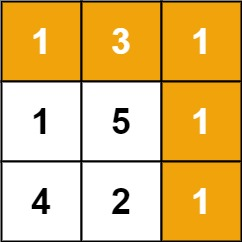# GeetCode Hub

Given a `m x n` `grid` filled with non-negative numbers, find a path from top left to bottom right, which minimizes the sum of all numbers along its path.

Note: You can only move either down or right at any point in time.

Example 1:```Input: grid = [[1,3,1],[1,5,1],[4,2,1]]
Output: 7
Explanation: Because the path 1 → 3 → 1 → 1 → 1 minimizes the sum.
```

Example 2:

```Input: grid = [[1,2,3],[4,5,6]]
Output: 12
```

Constraints:

• `m == grid.length`
• `n == grid[i].length`
• `1 <= m, n <= 200`
• `0 <= grid[i][j] <= 100`

class Solution { public int minPathSum(int[][] grid) { } }